# Transforming vectors with matrices#### Everything You Need in One Place

Homework problems? Exam preparation? Trying to grasp a concept or just brushing up the basics? Our extensive help & practice library have got you covered.#### Learn and Practice With Ease

Our proven video lessons ease you through problems quickly, and you get tonnes of friendly practice on questions that trip students up on tests and finals.#### Instant and Unlimited Help

Our personalized learning platform enables you to instantly find the exact walkthrough to your specific type of question. Activate unlimited help now!##### Intros
###### Lessons
1. Transforming vectors with matrices overview
##### Examples
###### Lessons
1. Finding the Transformed Vectors
Apply the transformation matrix $T$ to the following column vector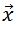to find the transformed vector:
1.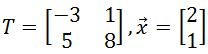2.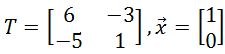3.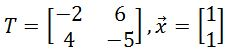4.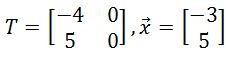5.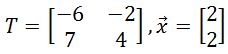2. Graphing the Transformed Vector
Plot the column vector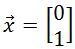on the graph. Then apply the transformation matrix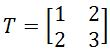to the column vector to find the transformed vector, and plot the transformed vector on the graph.
1. Plot the column vector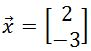on the graph. Then apply the transformation matrix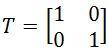to the column vector to find the transformed vector, and plot the transformed vector on the graph.
1. Plot the column vector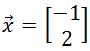on the graph. Then apply the transformation matrix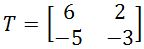to the column vector to find the transformed vector, and plot the transformed vector on the graph.
###### Topic Notes
In this lesson, we will learn how to transform column vectors with matrices. To transform a vector, we need to multiply the transformation matrix with that vector. This would result in a transformed vector, where the length or direction of the vector may be changed. We will take a look at some questions which involve transforming vectors, and then graph them to notice the changes between the normal vector and the transformed vector.
Letbe a column vector and $T$ be a transformation matrix. Then we can transform the column vectorby multiplying it with the transformation matrix $T$. In other words,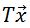is the transformed vector.